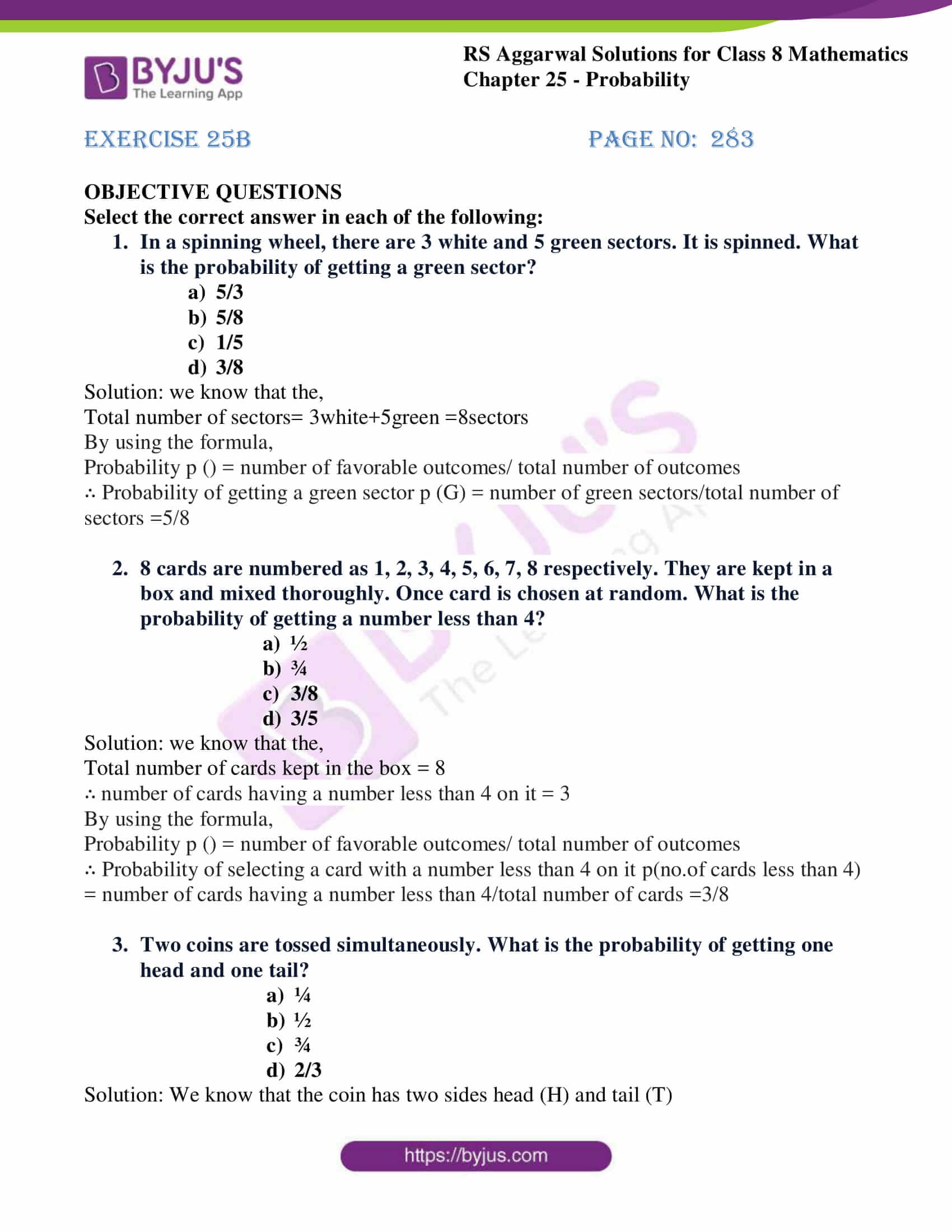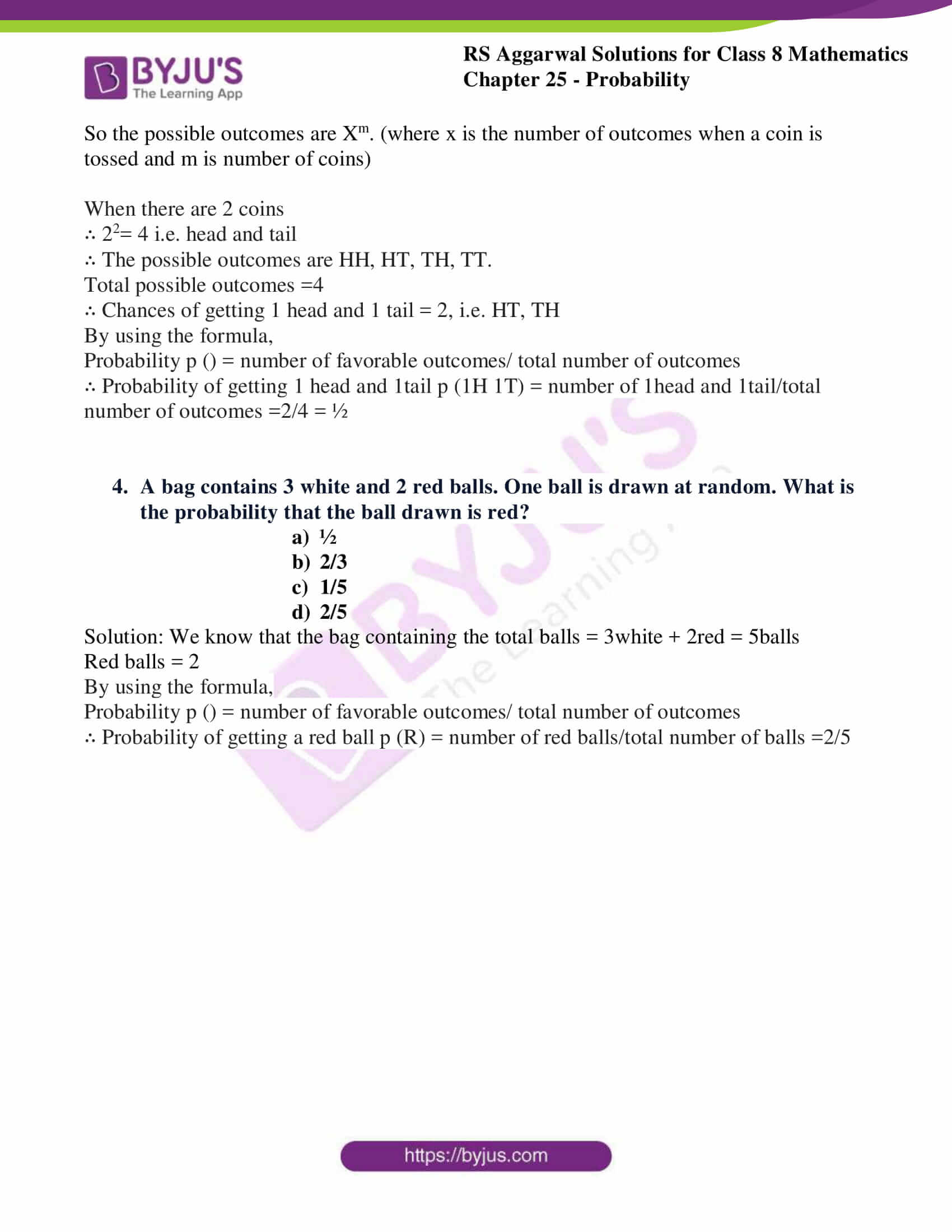# RS Aggarwal Solutions for Class 8 Maths Chapter 25 - Probability Exercise 25B

Students can refer and download RS Aggarwal Solutions for Class 8 Maths Chapter 25- Exercise 25B, Probability from the links provided below. The faculty members at BYJU’S have articulated the answers in an efficient manner to make students understand them faster while working on problems. In the solutions of the questions present in Exercise 25B, of RS Aggarwal Class 8 Maths, we shall brief all the concepts covered in Chapter 25.

## Download PDF of RS Aggarwal Solutions for Class 8 Maths Chapter 25- Probability Exercise 25B### Access Answers to RS Aggarwal Solutions for Class 8 Maths Chapter 25- Probability Exercise 25B

OBJECTIVE QUESTIONS

Select the correct answer in each of the following:

1. In a spinning wheel, there are 3 white and 5 green sectors. It is spinned. What is the probability of getting a green sector?

1. 5/3
2. 5/8
3. 1/5
4. 3/8

Solution: we know that the,
Total number of sectors= 3white+5green =8sectors
By using the formula,
Probability p () = number of favorable outcomes/ total number of outcomes
∴ Probability of getting a green sector p (G) = number of green sectors/total number of sectors =5/8

2. 8 cards are numbered as 1, 2, 3, 4, 5, 6, 7, 8 respectively. They are kept in a box and mixed thoroughly. Once card is chosen at random. What is the probability of getting a number less than 4?

1. ½
2. ¾
3. 3/8
4. 3/5

Solution: we know that the,
Total number of cards kept in the box = 8
∴ number of cards having a number less than 4 on it = 3
By using the formula,
Probability p () = number of favorable outcomes/ total number of outcomes
∴ Probability of selecting a card with a number less than 4 on it p(no.of cards less than 4) = number of cards having a number less than 4/total number of cards =3/8

3. Two coins are tossed simultaneously. What is the probability of getting one head and one tail?

1. ¼
2. ½
3. ¾
4. 2/3

Solution: We know that the coin has two sides head (H) and tail (T)
So the possible outcomes are Xm. (where x is the number of outcomes when a coin is tossed and m is number of coins)
When there are 2 coins
∴ 22= 4 i.e. head and tail
∴ The possible outcomes are HH, HT, TH, TT.
Total possible outcomes =4
∴ Chances of getting 1 head and 1 tail = 2, i.e. HT, TH
By using the formula,
Probability p () = number of favorable outcomes/ total number of outcomes
∴ Probability of getting 1 head and 1tail p (1H 1T) = number of 1head and 1tail/total number of outcomes =2/4 = ½

4. A bag contains 3 white and 2 red balls. One ball is drawn at random. What is the probability that the ball drawn is red?

1. ½
2. 2/3
3. 1/5
4. 2/5

Solution: We know that the bag containing the total balls = 3white + 2red = 5balls
Red balls = 2
By using the formula,
Probability p () = number of favorable outcomes/ total number of outcomes
∴ Probability of getting a red ball p (R) = number of red balls/total number of balls =2/5

### Access other Exercise of RS Aggarwal Solutions for Class 8 Maths Chapter 25- Probability

Exercise 25A Solutions 12 Questions

## RS Aggarwal Solutions for Class 8 Maths Chapter 25- Probability Exercise 25B

Exercise 25B of RS Aggarwal Class 8, Probability, This exercise mainly consists of objective questions related to the topics discussed earlier in Chapter 25. The RS Aggarwal Solutions can help the students practice diligently while learning the fundamentals as it provides all the answers to the questions from the RS Aggarwal textbook. Students are suggested to practice the problems on a regular basis which will help them excel in their exams and increase their overall percentage. Practicing as many times as possible helps in building time management skills and also boosts the confidence level to achieve high marks.